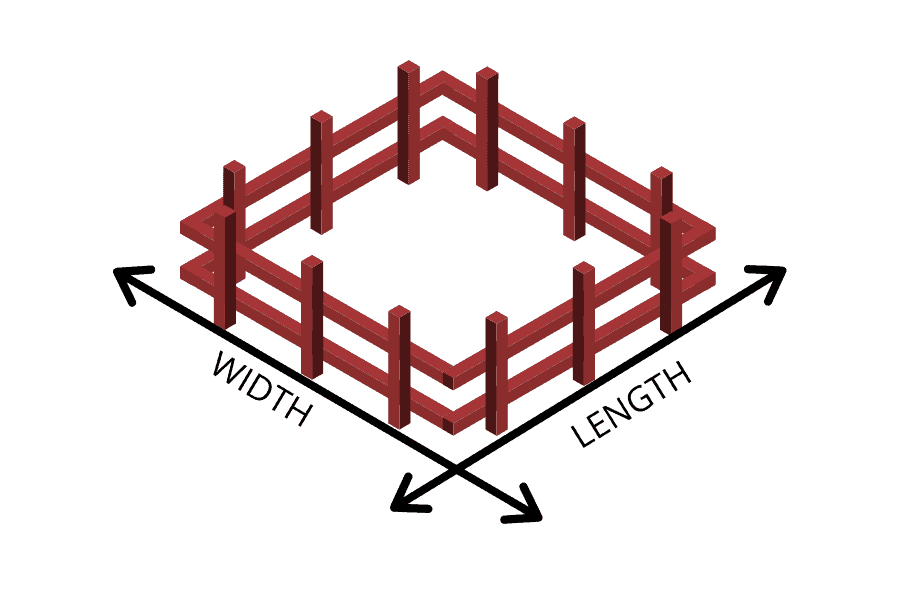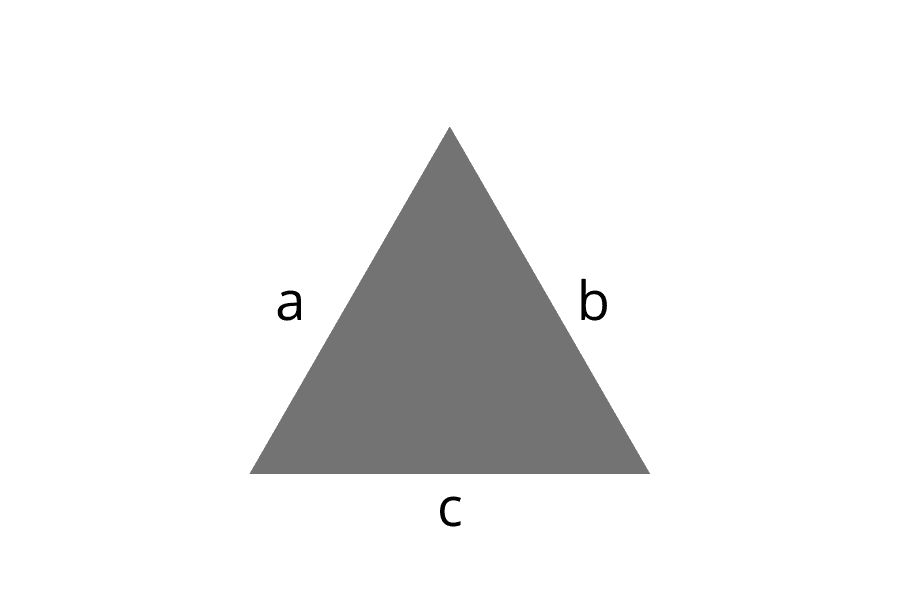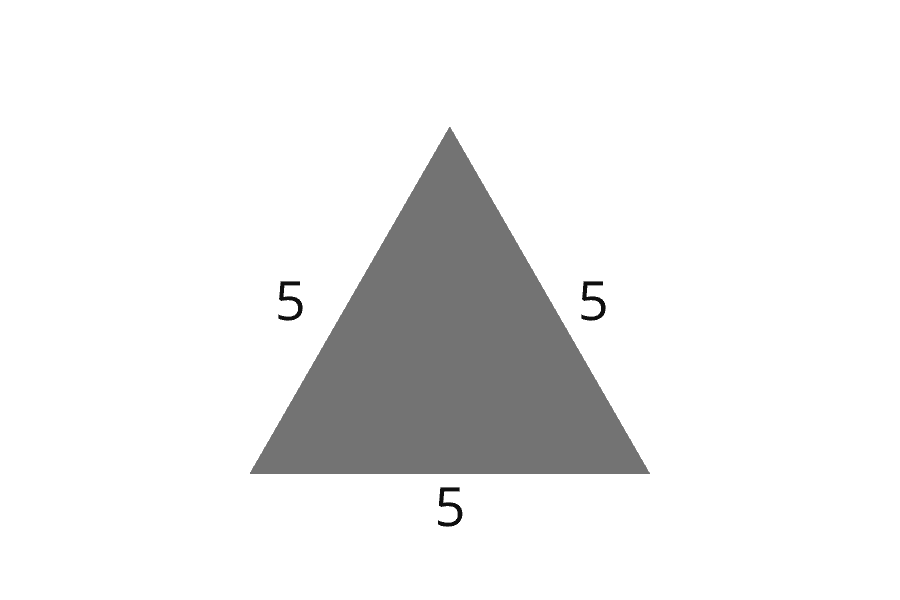# How to Find the Perimeter of a Triangle

In geometry, you’ll learn how to find the perimeter of many different shapes.

A perimeter is a path or the boundary that surrounds a shape. It measures the distance or length around a shape.

In math, the perimeter of a shape is the total length of the sides of a two-dimensional shape.

For example, think about building a fence around your yard. The fence will go around the perimeter of the yard. To figure out how much fencing you’ll need, you’ll need to measure your yard to see how long and how wide it is.## Perimeter of a Triangle

A triangle has three sides. The sides of the triangle are a, b, and c.

To find the perimeter of a triangle, you would add all sides together:

Perimeter = a + b + cIn this triangle, all sides are the same, so we would simply add all three sides together to get the perimeter.

P = 5 + 5 +5P = 15